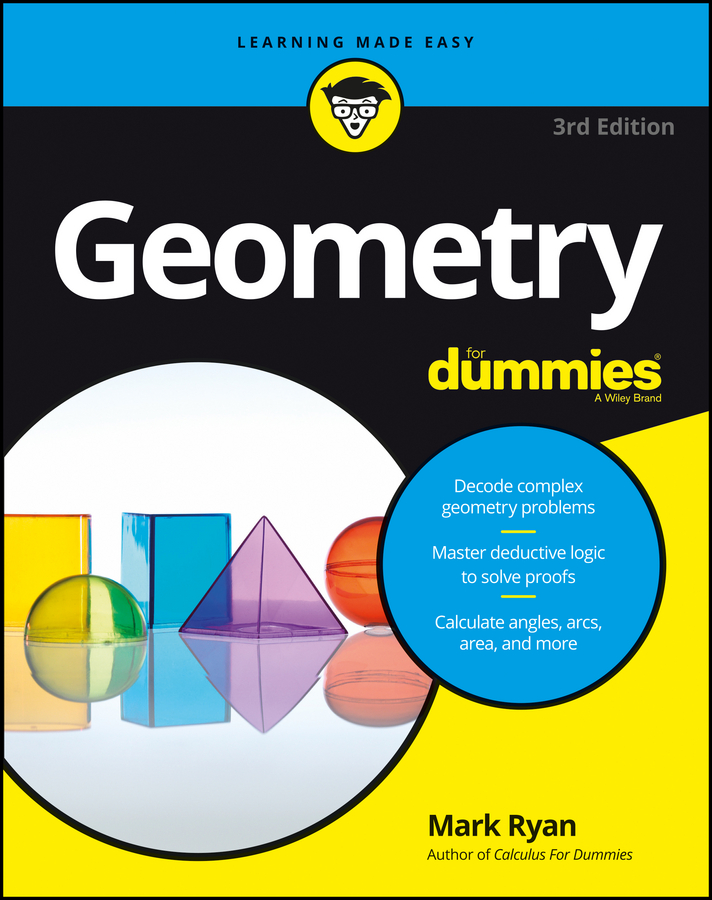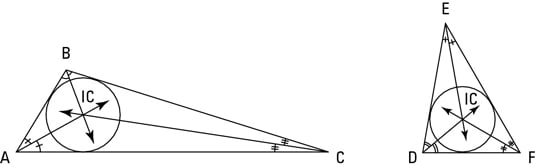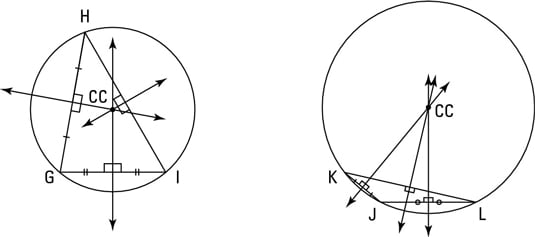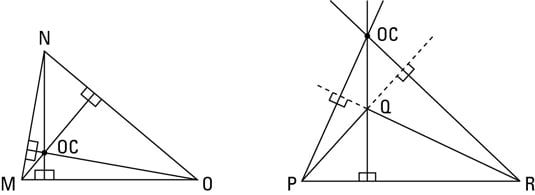##### Geometry For DummiesEvery triangle has three “centers” — an incenter, a circumcenter, and an orthocenter — that are located at the intersection of rays, lines, and segments associated with the triangle:

• Incenter: Where a triangle’s three angle bisectors intersect (an angle bisector is a ray that cuts an angle in half); the incenter is the center of a circle inscribed in (drawn inside) the triangle.

• Circumcenter: Where the three perpendicular bisectors of the sides of a triangle intersect (a perpendicular bisector is a line that forms a 90° angle with a segment and cuts the segment in half); the circumcenter is the center of a circle circumscribed about (drawn around) the triangle.

• Orthocenter: Where the triangle’s three altitudes intersect. The altitude of a triangle is a segment from a vertex of the triangle to the opposite side (or to the extension of the opposite side if necessary) that’s perpendicular to the opposite side; the opposite side is called the base.

## Finding the incenter

You find a triangle’s incenter at the intersection of the triangle’s three angle bisectors. This location gives the incenter an interesting property: The incenter is equally far away from the triangle’s three sides. No other point has this quality. Incenters, like centroids, are always inside their triangles.The above figure shows two triangles with their incenters and inscribed circles, or incircles (circles drawn inside the triangles so the circles barely touch the sides of each triangle). The incenters are the centers of the incircles. (Don’t talk about this “in” stuff too much if you want to be in with the in-crowd.)

## Finding the circumcenter

You find a triangle’s circumcenter at the intersection of the perpendicular bisectors of the triangle’s sides. This location gives the circumcenter an interesting property: the circumcenter is equally far away from the triangle’s three vertices.The above figure shows two triangles with their circumcenters and circumscribed circles, or circumcircles (circles drawn around the triangles so that the circles go through each triangle’s vertices). The circumcenters are the centers of the circumcircles.

You can see in the above figure that, unlike centroids and incenters, a circumcenter is sometimes outside the triangle. The circumcenter is

• Inside all acute triangles

• Outside all obtuse triangles

• On all right triangles (at the midpoint of the hypotenuse)

## Finding the orthocenter

Check out the following figure to see a couple of orthocenters. You find a triangle’s orthocenter at the intersection of its altitudes. Unlike the centroid, incenter, and circumcenter — all of which are located at an interesting point of the triangle (the triangle’s center of gravity, the point equidistant from the triangle’s sides, and the point equidistant from the triangle’s vertices, respectively), a triangle’s orthocenter doesn’t lie at a point with any such nice characteristics. Well, three out of four ain’t bad.But get a load of this: Look again at the triangles in the figure. Take the four labeled points of either triangle (the three vertices plus the orthocenter). If you make a triangle out of any three of those four points, the fourth point is the orthocenter of that triangle. Pretty sweet, eh?

Orthocenters follow the same rule as circumcenters (note that both orthocenters and circumcenters involve perpendicular lines — altitudes and perpendicular bisectors): The orthocenter is

• Inside all acute triangles

• Outside all obtuse triangles

• On all right triangles (at the right angle vertex)